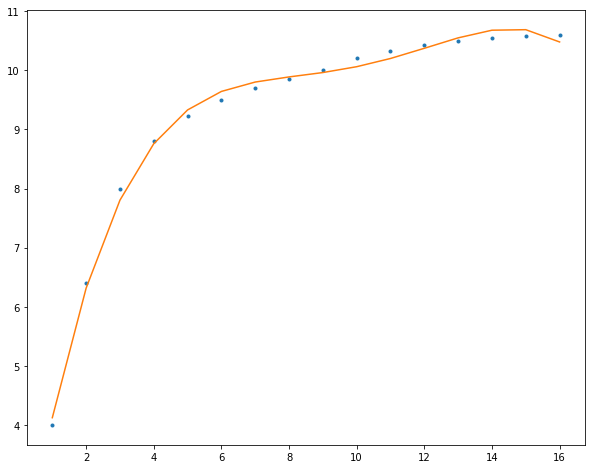# 曲线拟合

• 平滑

我们使用一个函数来尽可能的表示（即逼近）数据集中点的数据。

• 逼近

或者如前文所述，我们只想使用曲线拟合来平滑数据并改善绘图的外观。前两种情况下，数据的一般会有相当程度的误差或噪声。

如下所示，为线性拟合：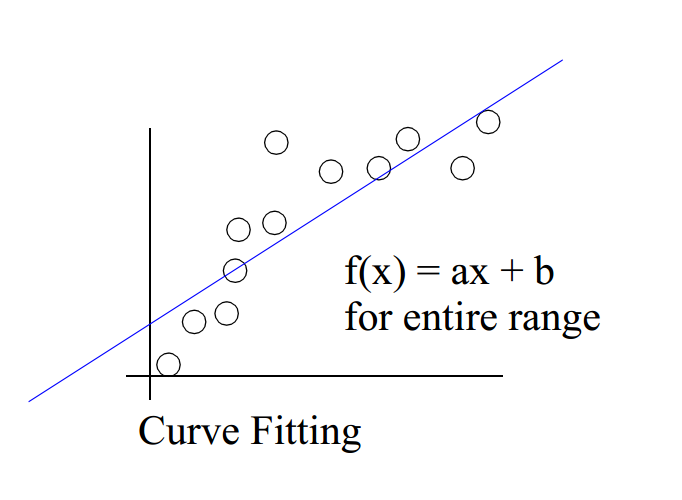• 插值

对于给定的数据集，产生一个或多个插值曲线通过数据集的每一个点。这种情况下，我们认为数据点很足够准确的。

如图所示，为线性插值：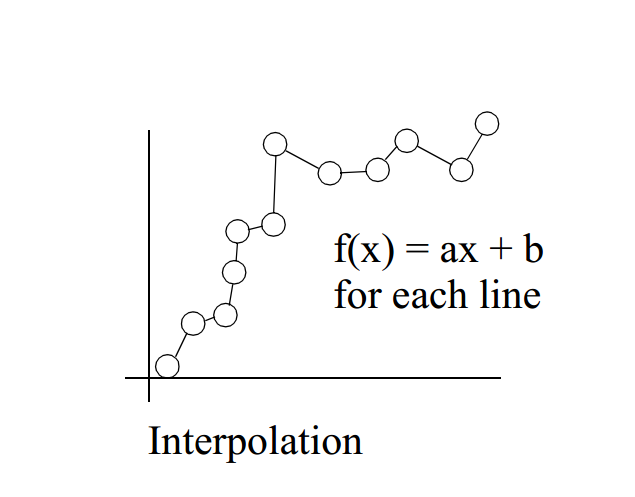# 多项式曲线拟合

## 建立模型——多项式曲线

$f(x)=a_n*x^n+a_{n-1}*x^{n-1}+···+a_2*x^2+a_1*x+a_0$

$f(x)=\sum_{i=0}^{n}{a_i*x^i}$

$n$为1时，即为线性函数$y=a*x+b$$n$为2时，则是二次函数(quadratic function)，$n$为3时，自然是三次函数（cubic function）。

$\left\{\begin{matrix} \hat{y_1}(x,w)=w_0+w_1*x_1+w_2*x_1^2+···+w_{m-1}*x_1^{m-1}+w_m*x_1^m \\ \hat{y_2}(x,w)=w_0+w_1*x_2+w_2*x_2^2+···+w_{m-1}*x_2^{m-1}+w_m*x_2^m \\ \vdots \\ \hat{y_{n-1}}(x,w)=w_0+w_1*x_{n-1}+w_2*x_{n-1}^2+···+w_{m-1}*x_{n-1}^{m-1}+w_m*x_{n-1}^m \\ \hat{y_n}(x,w)=w_0+w_1*x_n+w_2*x_n^2+···+w_{m-1}*x_n^{m-1}+w_m*x_n^m \end{matrix}\right.$

$\hat{Y}=XW$

$X=\begin{bmatrix} 1 & 1 & 1 & \cdots & 1\\ x_1 & x_2 & x_3 & \cdots & x_n \\ \vdots & \vdots & \vdots & \vdots & \vdots \\ x_1^m & x_2^m & x_3^m & \cdots & x_n^m \end{bmatrix}^T$

# 最小二乘法

1. 超定方程 $m>n$，且$A$$b$均已知，其中之一或者二者可能存在误差和干扰
2. 盲矩阵方程 均$b$已知，$A$未知
3. 欠定方程 $m$A$$b$均已知，但$x$为稀疏向量

## 普通最小二乘

$Ax=b+\Delta b\Rightarrow Ax=b_0$

$\mathop{min}_x ||\Delta b||^2=||Ax-b||^2_2=(Ax-b)^T(Ax-b)$

$\hat{x}_{LS}=arg\mathop{min}_x||Ax-b||^2_2$

$\phi = x^TA^TAx-x^TA^Tb-b^TAx+b^Tb$

$\phi$相对于$x$的导数，并令结果等于零，则有

$\frac{d\phi}{dx}=2A^TAx-2A^Tb=0$

$A^TAx=A^Tb$

• 超定方程($m>n$)列满秩时，即$rank(A)=n$

由于$A^TA$非奇异，所以方程有唯一解

$x_{LS}=(A^TA)^{-1}A^Tb$

• 对于不满秩（$rank(A)）的超定方程，则最小二乘解为

$x_{LS}=(A^TA)^\dagger A^Tb$

其中$\dagger$表示该矩阵的Moore-Penrose逆矩阵，即伪逆。

## Gauss-Markov定理

• 这里最佳的意思是指相较于其他估计量有更小方差的估计量，同时把对估计量的寻找限制在所有可能的线性无偏估计量中。
• 值得注意的是这里不需要假定误差满足独立同分布(iid)或正态分布，而仅需要满足零均值不相关同方差这三个稍弱的条件。

E\{e\}=0 \\ Cov\{e\}=E\{ee^T\}=\sigma^2I \\

## 最小二乘法与最大似然解

$f(e)=\frac{1}{\pi^m|\Gamma_e|}exp[-(e-\mu_e)^T{\Gamma_e}^{-1}(e-\mu_e)]$

$f(e)=\frac{1}{(\pi\sigma^2)^m}exp(-\frac{1}{\sigma^2}e^Te)=\frac{1}{(\pi\sigma^2)^m}exp(-\frac{1}{\sigma^2}||e||^2_2)$

$L(e)=logf(e)=-\frac{1}{\pi^m\sigma^{2(m+1)}||e||^2_2}=-\frac{1}{\pi^m\sigma^{2(m+1)}}||Ax-b||^2_2$

$\hat{x}=arg \mathop{max}_{x}\frac{-1}{\pi^m\sigma^{2(m+1)}}||Ax-b||^2_2=arg\mathop{min}_x\frac{1}{2}||Ax-b||^2_2=\hat{x}_{LS}$

# Python实现

Python的numpy模块已经实现了很多数值函数，自然也包括了最小二乘法numpy.linalg.lstsq，并且numpy将其封装成了polyfit函数，然后配合poly1d函数，即可完成基于最小二乘法的多项式曲线拟合。

2阶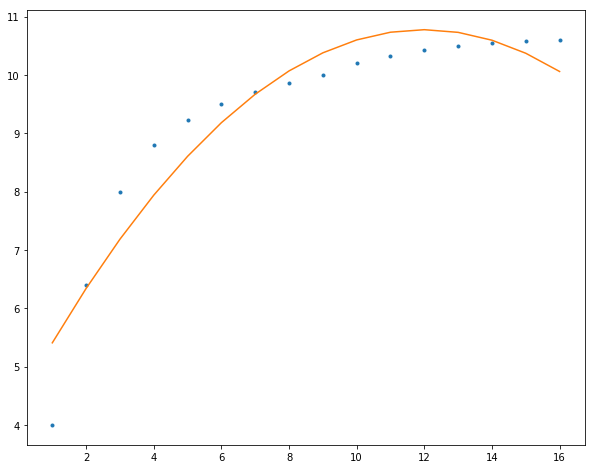3阶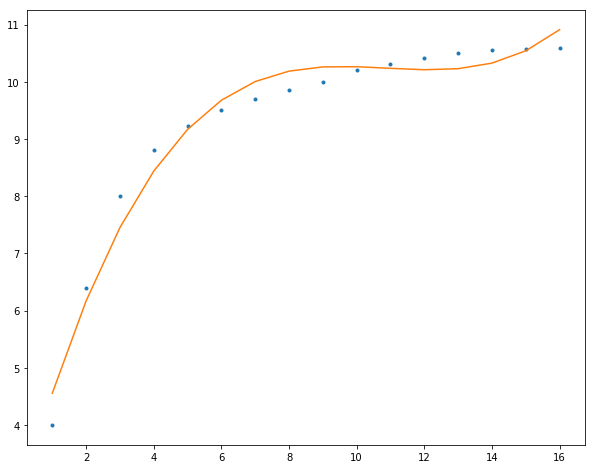4阶